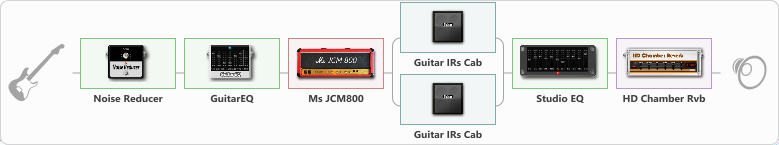# JCM800[ Studio Stack ]

Discussion in 'ToneLib-GFX presets' started by Jean C.Guion, Jul 24, 2020.

1. JCM800[ Studio Stack ]

Preset name: JCM800[ Studio Stak ]

Fully maxxed out jcm800....pre-Q'd and then run thru a full stack before running thru a sharply tuned studio Eq...lays into the mix very nicely..adjust amp gain using guitar Eq pedal output volume...

Effects chain:Effect: "Noise Reducer" (Dynamics / Filter), active - "yes"
{
"Sens" = 36
"Mode" = Soft
}

Effect: "GuitarEQ" (Dynamics / Filter), active - "yes"
{
"160 Hz" = -1
"400 Hz" = -1
"800 Hz" = 4
"1.6 kHz" = -1
"3.2 kHz" = -1
"6.4 kHz" = -3
"12 kHz" = -5
"Level (dB)" = -2
}

Effect: "Ms JCM800" (Amp simulators), active - "yes"
{
"Gain" = 100
"Bass" = 100
"Middle" = 100
"Treble" = 100
"Presence" = 100
"Master" = 100
"Level (dB)" = 0
}

Effect: "Splitter" (Dynamics / Filter), active - "yes"
{
"A-Bypass" = Off
"A-Pan" = 0
"A-Level" = 55
"B-Bypass" = Off
"B-Pan" = 0
"B-Level" = 55

'A' branch:
{

Effect: "Guitar IRs Cab" (Cabinets), active - "yes"
{
"Model" = Marshall 1960A (4x12")
"Mic Position" = Middle
"Mic Distance" = Far
"Low Cut (Hz)" = 98
"Hi Cut (kHz)" = 4.0
"Mix" = 100
"Level (dB)" = 0
}
}
'B' branch:
{

Effect: "Guitar IRs Cab" (Cabinets), active - "yes"
{
"Model" = Marshall 1960b (4x12")
"Mic Position" = Middle
"Mic Distance" = Far
"Low Cut (Hz)" = 89
"Hi Cut (kHz)" = 4.0
"Mix" = 100
"Level (dB)" = 0
}
}
}

Effect: "Studio EQ" (Dynamics / Filter), active - "yes"
{
"31 Hz" = -15
"62 Hz" = -11
"125 Hz" = -1
"250 Hz" = -7
"500 Hz" = -4
"1 kHz" = -9
"2 kHz" = -1
"4 kHz" = 1
"8 kHz" = -12
"16 kHz" = -12
"Level (dB)" = 0
}

Effect: "HD Chamber Rvb" (Reverberation), active - "yes"
{
"Time" = 3.4
"PreLPF" = 64
"PreDelay" = 142
"HiDamp" = 50
"LoGain" = 1.2
"Mix" = 42
}

Note: You will need to download and install the ToneLib-GFX software to use the preset.

File size:
1.1 KB
Views:
3,000